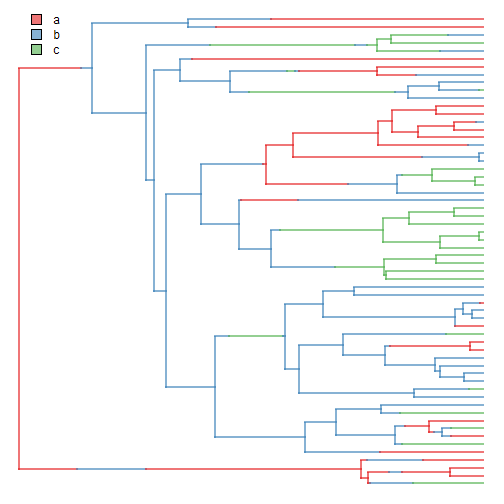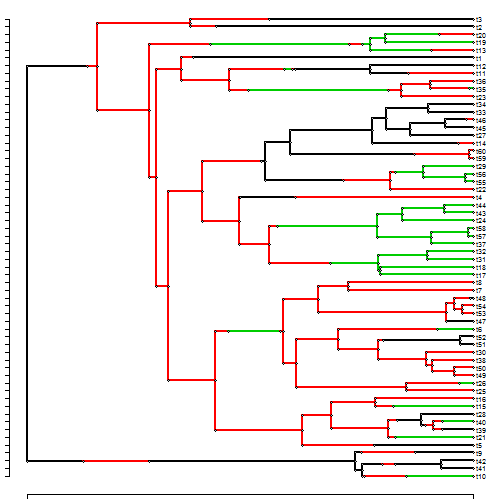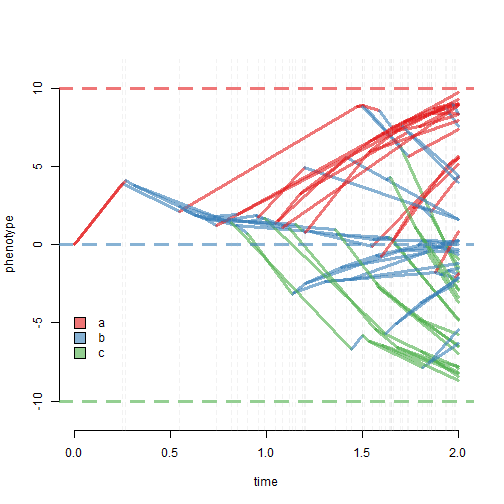## Monday, March 13, 2017

### Visualizing simulated Ornstein-Uhlenbeck evolution on a phylogeny in which the OU regime changes along edges of the tree using singleton nodes

One of the utilities of the function `multiOU` that I posted yesterdy featuring a difference equation approximation of the OU model is that it can easily be used to visualize the evolutionary process by permitting the user to easily retain the states simulated for internal nodes.

A limitation of this is that the evolution along internal edges can include multiple, different regimes.

In the following, I show how to simulate & visualize multi-regime OU in which the regime changes along edges through the use of singleton nodes. With singleton nodes we can break edges of the tree into a set of new edges, each of which has only one regime. I'll use semi-transparent colors to make it easier to visualize the evolutionary process using our traitgram projection.

``````library(phytools)
set.seed(100)
cols<-setNames(sapply(c("#e41a1c","#377eb8","#4daf4a"),
make.transparent,alpha=0.6),letters[1:3])
tree<-pbtree(n=60,scale=2)
Q<-matrix(c(-1,1,0,1,-2,1,0,1,-1),3,3,
dimnames=list(letters[1:3],letters[1:3]))
tree<-sim.history(tree,Q,anc="a")
``````
``````## Done simulation(s).
``````
``````plot(tree,colors=cols,ftype="off",lwd=2)
tmp<-markChanges(tree,plot=FALSE) ## for later
``````Next, convert our object to a tree with singletons. To that object, we will also add some elements & a class attribute so that `multiOU` will know how to treat the object:

``````obj<-map.to.singleton(tree)
obj\$maps<-as.list(obj\$edge.length)
for(i in 1:length(obj\$maps)) names(obj\$maps[[i]])<-names(obj\$maps)[i]
class(obj)<-c("simmap","phylo")
## here's our tree with singletons
par(cex=0.6)
plotTree.singletons(obj) ## the colors are different
``````OK, now let's simulate on the tree with singletons:

``````sig2<-setNames(c(1,1,1),letters[1:3])
alpha<-setNames(c(2,2,2),letters[1:3])
theta<-setNames(c(10,0,-10),letters[1:3])
x<-multiOU(obj,alpha,sig2,theta,internal=TRUE,dt=1/10000*2)
phenogram(obj,x,ftype="off",colors=cols,ylim=c(-11,11),
xlim=c(0,2),lwd=3)
abline(h=c(10,0,-10),lty="dashed",col=cols,lwd=3,lend=2)
abline(v=tmp[,"x"],lwd=1,lty="dashed",col=make.transparent("grey",0.2))1.